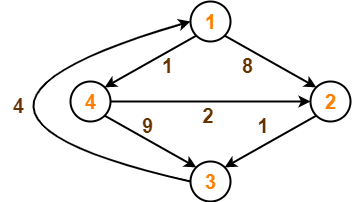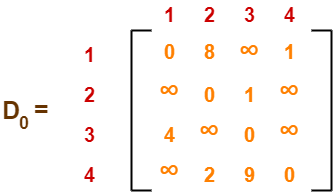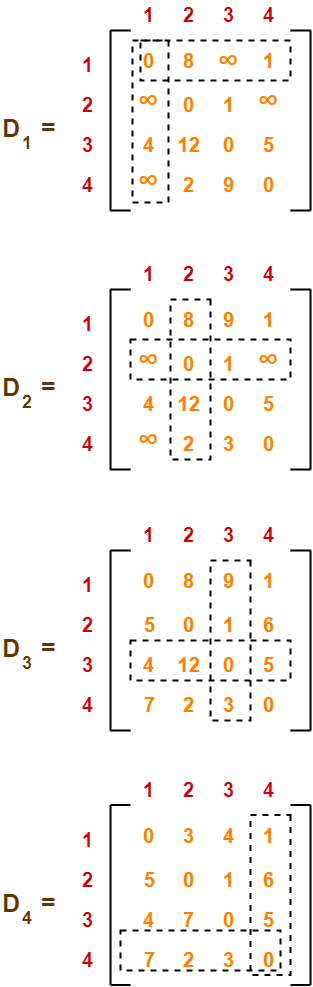# Floyd Warshall Algorithm | Example | Time Complexity

## Floyd Warshall Algorithm-

• Floyd Warshall Algorithm is a famous algorithm.
• It is used to solve All Pairs Shortest Path Problem.
• It computes the shortest path between every pair of vertices of the given graph.
• Floyd Warshall Algorithm is an example of dynamic programming approach.

Floyd Warshall Algorithm has the following main advantages-

• It is extremely simple.
• It is easy to implement.

### Algorithm-

Floyd Warshall Algorithm is as shown below-

```Create a |V| x |V| matrix               // It represents the distance between every pair of vertices as given

For each cell (i,j) in M do-

if i = = j

M[ i ][ j ] = 0                 // For all diagonal elements, value = 0

if (i , j) is an edge in E

M[ i ][ j ] = weight(i,j)       // If there exists a direct edge between the vertices, value = weight of edge

else

M[ i ][ j ] = infinity          // If there is no direct edge between the vertices, value = ∞

for k from 1 to |V|

for i from 1 to |V|

for j from 1 to |V|

if M[ i ][ j ] > M[ i ][ k ] + M[ k ][ j ]
M[ i ][ j ] = M[ i ][ k ] + M[ k ][ j ]```

### Time Complexity-

• Floyd Warshall Algorithm consists of three loops over all the nodes.
• The inner most loop consists of only constant complexity operations.
• Hence, the asymptotic complexity of Floyd Warshall algorithm is O(n3).
• Here, n is the number of nodes in the given graph.

### When Floyd Warshall Algorithm Is Used?

• Floyd Warshall Algorithm is best suited for dense graphs.
• This is because its complexity depends only on the number of vertices in the given graph.
• For sparse graphs, Johnson’s Algorithm is more suitable.

## Problem-

Consider the following directed weighted graph-Using Floyd Warshall Algorithm, find the shortest path distance between every pair of vertices.

## Solution-

### Step-01:

• Remove all the self loops and parallel edges (keeping the lowest weight edge) from the graph.
• In the given graph, there are neither self edges nor parallel edges.

### Step-02:

• Write the initial distance matrix.
• It represents the distance between every pair of vertices in the form of given weights.
• For diagonal elements (representing self-loops), distance value = 0.
• For vertices having a direct edge between them, distance value = weight of that edge.
• For vertices having no direct edge between them, distance value = ∞.

Initial distance matrix for the given graph is-### Step-03:

Using Floyd Warshall Algorithm, write the following 4 matrices-To learn how to write these matrices, watch this video here.

The last matrix D4 represents the shortest path distance between every pair of vertices.

### Remember-

• In the above problem, there are 4 vertices in the given graph.
• So, there will be total 4 matrices of order 4 x 4 in the solution excluding the initial distance matrix.
• Diagonal elements of each matrix will always be 0.

To gain better understanding about Floyd Warshall Algorithm,

Watch this Video Lecture

Next Article- Knapsack Problem

Get more notes and other study material of Design and Analysis of Algorithms.

Watch video lectures by visiting our YouTube channel LearnVidFun.

SummaryArticle Name
Floyd Warshall Algorithm | Example | Time Complexity
Description
Floyd Warshall Algorithm is a dynamic programming algorithm used to solve All Pairs Shortest path problem. Floyd Warshall Algorithm Example Step by Step. The time complexity of Floyd Warshall algorithm is O(n3).
Author
Publisher Name
Gate Vidyalay
Publisher Logo